# Dynamics of the Firm

The model’s outcomes for firm-level labor demand through hiring, firing, and layoff can be illustrated through the optimal decisions of the planner’s problem for a firm. These conditions highlight that firm expansion occurs gradually over time through variations in the number of vacancies posted by the firm and the matching probability of each vacancy.

The planner’s problem can be solved given a vector M = (^b …,ựn) of mul­tipliers on the resource constraint (1.13), which correspond to the planner’s value of a worker in n aggregate states. The first-order conditions determine optimal policies for s,a,m, and V for a given level of productivity and firm size L. The first or­der conditions for m and V relate the inter-temporal and intra-temporal tradeoffs of recruitment intensity for firms. The optimal recruitment policy in a stationary equi­librium features a declining match probability as L increases to the firm’s optimal size.

Suppressing the state (x, z) for policy functions, the first-order condition with respect to m gives: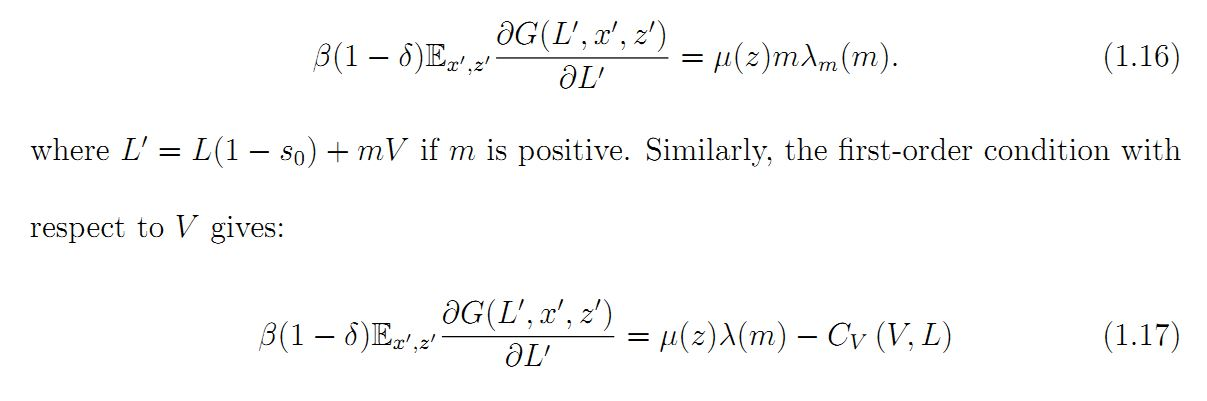Combining (1.16) and (1.17) gives the intra-temporal optimality condition for hiring, describing the trade-off to the planner of increasing vacancies and increasing the matching probability for the firm. Intuitively, the planner equates the marginal cost to the firm of hiring another worker by opening more vacancies with the marginal cost of hiring an additional worker by increasing the matching probability of vacancies through more valuable contracts: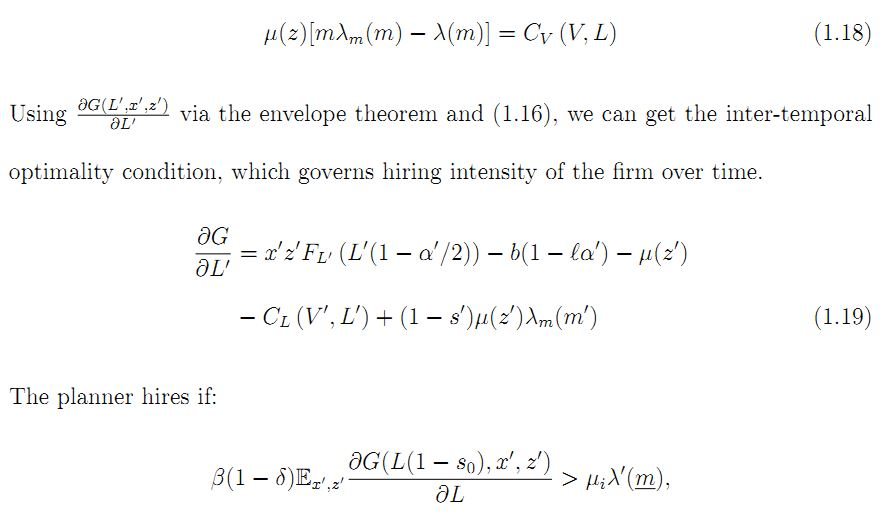where m is the value of m for which A(m) = 0. If this inequality is satisfied, (1.18) characterizes the optimal vacancy postings V*(m(L, x, z)) for a given matching prob­ability m which satisfies (1.16) with (1.19) substituted inside the expectation term.

Figure 1.8 shows the vacancies posted and number of hires (mV) for a firm of a given productivity level as a function of its current workforce. The increasing gap between the number of vacancies posted and the number of hires with labor L reflects the declining matching probability used by the firm as it reaches maturity. Since vacancy posting costs are convex, the firm spaces hiring over time.

The optimal choice of a and s can also be solved from the first-order conditions of the firm’s social surplus problem.

An alternative way to write this condition is to consider the planner’s (firm’s) ideal labor utilization today, L. The optimal labor utilization solves: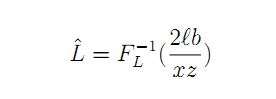. Since FL is positive and decreasing, its inverse is also decreasing. Thus, labor utilization L is increasing in productivity xz and decreasing in the share of leisure gained by moving from full-time to part-time work, ữ). Intuitively, if the marginal product of labor in production today is high, the planner wants to allocate worker time to production and away from leisure. When the current marginal product of labor is low enough, the planner would like to move workers to part-time as the gains from added leisure are higher than the gains in output for the marginal worker. Writing L
in terms of L and a and assuming an interior solution, the optimal part-time usage

If the optimal labor utilization L is outside of the interval (L/2, L), then a* is a corner solution. When interior, part-time use is increasing in the leisure gained from part-time work, ữ). Part-time use is decreasing in productivity, xz. We can also see that part-time usage is increasing in L, all else equal. It should be noted that the part-time decision is independent of future values of the firm, but the current size L for the firm is a function of past size and productivity as well as the future value of these workers to the firm. Thus, actual part-time utilization by firms depends on the difference between their actual size and their ideal current size, and it is not clear that part-time usage will be increasing or decreasing in firm size categories in the stationary distribution.

The first-order condition with respect to s is: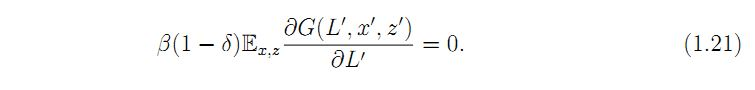If the optimal labor utilization L is outside of the interval (L/2, L), then a* is a corner solution. When interior, part-time use is increasing in the leisure gained from part-time work, ữ). Part-time use is decreasing in productivity, xz. We can also see that part-time usage is increasing in L, all else equal. It should be noted that the part-time decision is independent of future values of the firm, but the current size L for the firm is a function of past size and productivity as well as the future value of these workers to the firm. Thus, actual part-time utilization by firms depends on the difference between their actual size and their ideal current size, and it is not clear that part-time usage will be increasing or decreasing in firm size categories in the stationary distribution.

The first-order condition with respect to s is: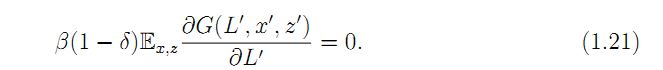Again, if the solution is interior then (1.21) holds with equality. If the expected value of labor tomorrow is high, then the planner will not separate with workers.

From the first order conditions for a and s, the planner will utilize part-time labor when the value of current productivity is low. If the marginal value of its future labor force is also low, the planner will separate with workers at the end of the period.

Figure 1.9 shows the part-time probability and separation rate for a firm at two different productivity levels (high and low). The part-time employment rate (red line) and separation probability (light gray line) of the firm are the two upward-sloping lines at each productivity level. The slope of the part-time percentage is steeper than the separation rate. For a given size, the part-time utilization rate is increasing faster than the separation rate as the size of the shock increases. In general, the part-time rate can be positive even if the firm is not separating (it may even be expanding), depending on parameters. A high productivity firm initially at size L which realizes a low productivity shock would have policy functions of the solid lines in Figure 1.9. The black points on the part-time and layoff lines indicate the firm’s choice of part­time usage that period, as well as the fraction of its workforce it will layoff at the end of the period. Upon realization of the shock from high to low productivity, the firm would use part-time employment to decrease its labor utilization in the current period. After production, the firm would separate with 35% of its workers, starting the next period with 65% of its original labor force.

While the policy functions and first-order conditions give intuition on the factors that affect firm-level decisions for employment and part-time utilization, the actual dynamics of the firm requires tracking of a firm’s state variable over time. The firm’s state variables (L, x, z ) move according to the transition processes for productivity and the law of motion for firm size (L = L(1 — s) + mV) dictated by the policy functions. In Figure 1.10, I plot the simulation of an individual firm subject to idiosyncratic and aggregate shocks over a 20 year period using parameters from the calibrated model. The firm grows upon entry and fluctuates with realized productivity shocks. Steep declines in the firm’s size are the result of layoffs, while steady declines result from inaction in hiring or firing by the firm due to the exogenous separation of workers from the firm (at rate s0 ). It is clear from the figure that part­time employment is volatile at the firm level and is used in conjunction with layoffs.